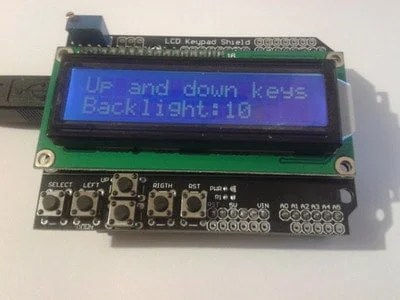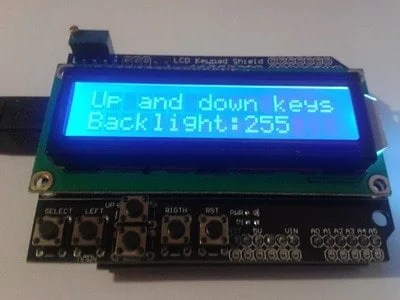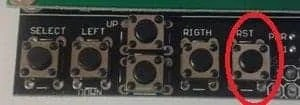# Testing LCD keypad Shield for Arduino

Recently I’ve got an Arduino LCD keypad shield. Haven’t decided yet where will it be used. But why not to plug it to Arduino board and see it working. The shield was initially introduced by DFRobot who has some cool open-source stuff including robotics related.This LCD keypad shield is a cheap and convenient solution for adding 2×16 LCD and five push buttons (+1 reset) to Arduino design. LCD here is interfaced using 4-bit mode and occupies 4 (D4), 5 (D5), 6(D6), 7(D7), 8(RS), 9(E) and ten digital pins. Pin 10 is used to control LCD backlight through transistor key. All five buttons are connected to single Analog pin 0 using a resistor-based voltage divider. This lets us keep other pins for general use. The shield is designed to work with 5V based boards.

Since Arduino already has an LCD library implemented in the core, it is easy to start using it right away by initializing LCD with a simple command:

LiquidCrystal lcd(8, 9, 4, 5, 6, 7);

But since there is a dedicated library for this shield things become even simpler. All you need is to download one of the libraries. I suggest using LCDKeypad.zip which simplifies usage of LCD a bit and also implements key reading.If we look at keys connected to analog pin 0 we will see that when any of the keys is pressed we get a simple voltage divider. With all known resistor values and supply voltage we can calculate the voltage drop, and so ADC reading for each key. In the library, there are ADC ranges used to minimize error due to the variation of resistor values. Actually, you even don’t need a library for that since there is only one loop used to test the button. Let’s build a simple program where we would be able to adjust LCD backlight brightness:

 `#include ` `#include ` `//contrast step` `#define DELTA 10` `LCDKeypad lcd;` `//initial backlight` `unsigned ``char` `bckl=150;` `void` `setup(){` ` ``pinMode(10, OUTPUT);` ` ``analogWrite(10, bckl);` ` ``lcd.begin(16,2);` ` ``lcd.clear();` ` ``lcd.print(``"    Aadjust"``);` ` ``lcd.setCursor(0,1);` ` ``lcd.print(``"   Backlight"``);` ` ``delay(1000);` `}` `void` `loop(){` ` ``int` `btn;` ` ``lcd.clear();` ` ``lcd.print(``"Up and down keys"``);` ` ``lcd.setCursor(0,1);` ` ``lcd.print(``"Backlight:"``);` ` ``lcd.print(bckl);` `//infinite loop of setting backlight` ` ``while``(1)` ` ``{` ` ``//wait for button` ` ``lcd.blink();` ` ``while` `((btn=lcd.button())==KEYPAD_NONE)` ` ``{` ` ``}` ` ``delay(50);` ` ``lcd.noBlink();` ` ``//wait for button release` ` ``while``(lcd.button()!=KEYPAD_NONE)` ` ``{` ` ``}` ` ``//adjust the backlight` ` ``if` `(btn==KEYPAD_UP)` `    ``{` `      ``if` `((0xFF-DELTA)>=bckl)` `        ``bckl +=DELTA;` `      ``else` `bckl = 0xFF;` `    ``}` `    ``else` `if` `(btn==KEYPAD_DOWN)` `    ``{` `      ``if` `(DELTA<=bckl)` `        ``bckl -= DELTA;` `      ``else` `bckl = 0;` `    ``}` ` ``lcd.setCursor(0,1);` `//clear second LCD line` ` ``lcd.print(``"                "``);` ` ``lcd.setCursor(0,1);` ` ``lcd.print(``"Backlight:"``);` ` ``lcd.print(bckl);` ` ``//set new backlight` ` ``analogWrite(10, bckl);` ` ``}` ` ``}`

As you can see backlight of LCD is changed by simply sending PWM signal to pin 10 of Arduino. The minimum value is 0 which means the backlight of OFF and the maximum is 255 when LEDs are driven with a constant voltage. Here is the result of PWM set to 10 and 255 respectively:The last thing I would like to mention that this shield has an awkward Reset button position. It is placed next to five user buttons that can be used as menu navigation keys and other purposes. The reset button is aligned dangerously close to user keys and can be accidentally pressed during operation.Probably it would be better to place it anywhere else on PCB. Overall this is a great and robust shield which works fluently. It is up to you whether to use a library or achieve the same result with standard Arduino libraries. It shouldn’t be a problem to put this stacked assembly into a compact enclosure.

1. Jim Brown

Yep it’s a nice shield, but with it using pins 4-10 it’s a huge pity that pins 11, 12 and 13 aren’t broken out. Only pins 3 and 4 are available to the user so it’s very limiting.

2. Jim Brown

Haha cancel that previous comment: I just realised that the pins broken out at top-right include 11-13. Numbering from the right, those holes are 0, 1, 2, 3 (which I knew) and 11, 12 and 13 (which I just beeped out with my Fluke).

3. Yeah, all unused pins are broken out.

4. submarine

Thx to Jim,
how about the lower pin hole, are they represent A0-A5?

5. A1 to A5 can be used as there are holes. A0 is used by buttons.

6. Submarine

Woo, that’s cool. Thx. just wonder why the shield give holes instead of pin holes.

7. Submarine

Is it possible to use this shield on raspberry pi?
By connecting to the gpio.

8. Why not. Once you get RasPi talking to Arduino with USART, SPI or I2C you can do many crazy stuff.

9. Submarine

Yeah… Good pieces of DIY project

10. Gunni

Is there any way that the bckl value to be stored and be changeble, so if we reset the arduino will remember the value that we gave

11. Use EEPROM for that.

This site uses Akismet to reduce spam. Learn how your comment data is processed.